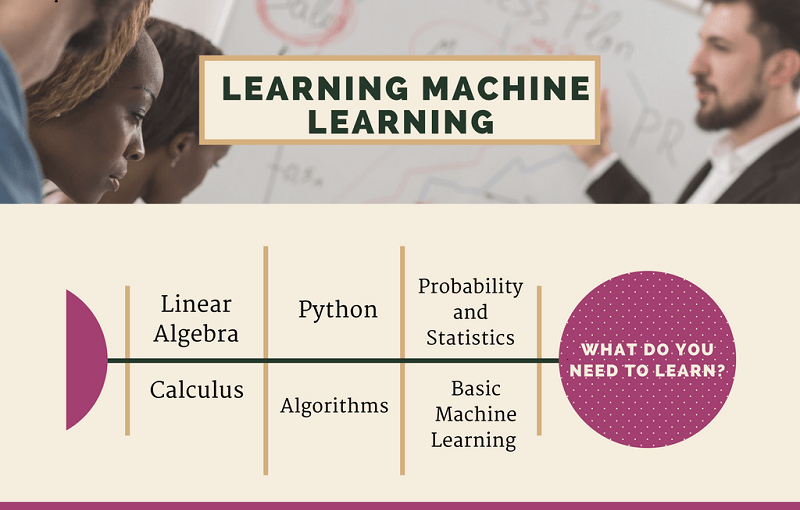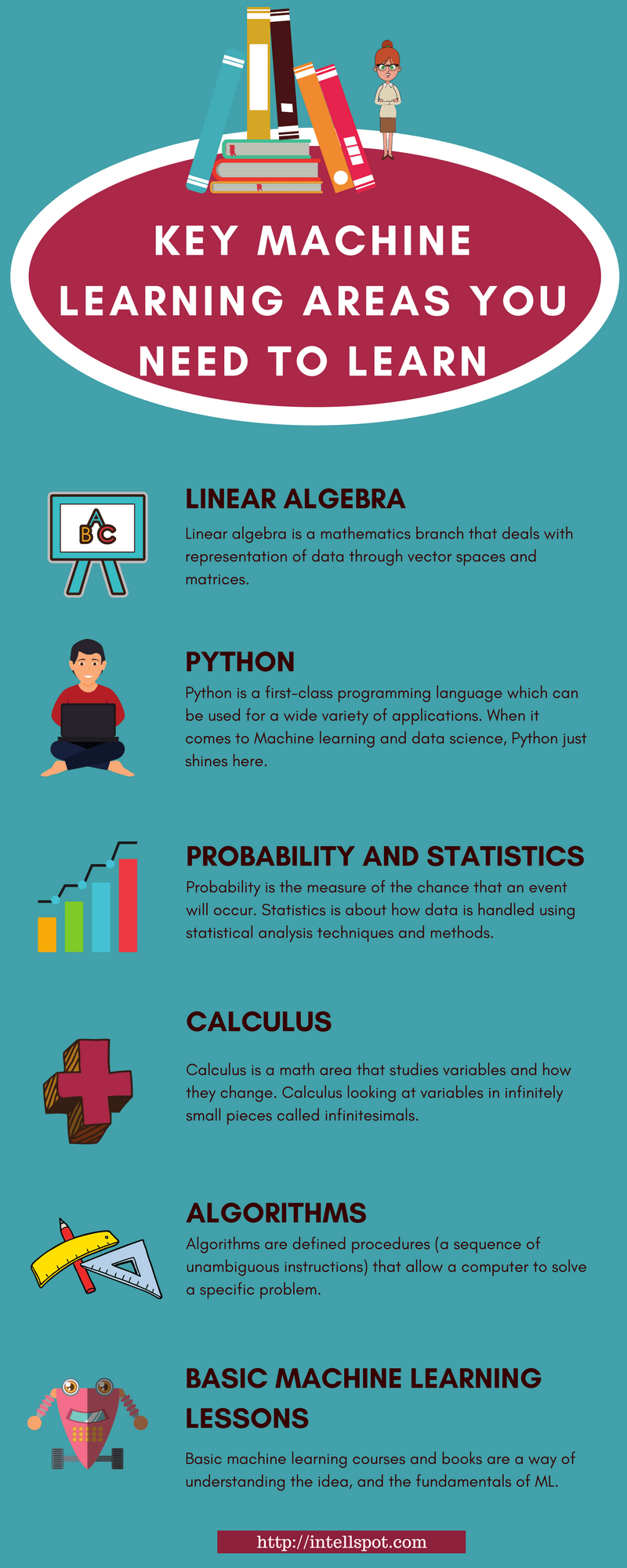Best Ways to Learn Machine Learning

As you have come to the point “What are the best ways to learn Machine Learning (ML)?”, you know ML is a critical skill and career path today.

Learning ML involves understanding maths, statistics, programming languages (such as Python and R), algorithms and etc.

Nowadays, there are many easy, fast, affordable or free ways to learn Machine Learning for beginners and advanced learners. You can find online courses, books, interactive lessons, websites, and places to gain and share knowledge.

• What is Machine Learning?
• Best ways to learn: Python, Probability, Algorithms, Calculus, Linear Algebra, and ML as a whole.

What is Machine Learning?

Machine Learning (ML) is a science of giving computers the ability to “learn” (i.e., perform a given task) and to take actions like humans do.

Simply put, it is a way of teaching computers to make predictions based on some data.

ML is based on the concept that computers can learn from data, identify patterns, trends and make some kind of decisions with minimal or no human intervention.

For that purpose, ML uses a set of algorithms and a variety of statistical techniques.

ML consists of two main distinctions: supervised and unsupervised learning algorithms. For more details see our post supervised vs unsupervised learning.

What do you need to learn?In short, learning ML includes learning linear algebra (e.g. simple linear regression), probability theory, calculus, Graph theory, programming languages, essential algorithms ( e.g. machine learning algorithms for classification), playing with datasets and etc.

For your convenience, I collected some best ways to learn Machine Learning grouped according to the key ML fields.

1. Learn Linear Algebra

What is linear algebra?

Linear algebra is a mathematics branch that deals very well with the representation of data through vector spaces and matrices. In other words, in linear algebra, data is represented as linear equations.

Linear Algebra is based on continuous data rather than discrete data. See our post discrete vs continuous data.

Why do I need to learn linear algebra for ML?

Linear algebra is a pillar of Machine Learning. Knowing linear algebra will give you some critical ML abilities such as reading and writing vector and matrix notation, describing operations on data, adding, subtracting, and multiplying vectors, and etc.

What are some best ways to learn linear algebra?

2. Learn Python

What is Python?

Python is a first-class programming language which can be used for a wide variety of applications. When it comes to Machine learning and data science, Python just shines here.

Why do I need to learn Python for ML?

It is the most popular programming language in machine learning by far.

There are many reasons for that. First, it is very easy to learn and to implement. Second, Python shines in terms of its easy syntactical character in comparison to other languages. Additionally, Python has an enormous set library which can be easily used for ML (such as NumPy, Scikit Learn, SciPy, etc).

If you are an ML beginner who starting from scratch, Python is the easiest language for learning in a short time.

What are some best ways to learn Python?

3. Learn Probability and Statistics

Probability and statistics are key areas of math that deal with data collection and data analysis.

What are Probability and Statistics?

Probability is the measure of the chance that an event will occur. On the other hand, the statistics is more about how data is handled using different statistical analysis techniques and methods.

Typically, these two fields go hand in hand and you need to study the both of them together.

Why do I need to learn Probability and Statistics?

To understand why and how machine learning algorithms work, you need to understand how probability and statistics work. Also, probability and statistics play a crucial role in expanding research and data insight.

Moreover, they help you explain the choices made by many machine learning models and the output of your algorithms. Another reason: probability and statistics determine what types of data analysis is required.

Best ways to learn Probability and Statistics:

4. Learn Calculus

What is Calculus?

Calculus is a math area that studies variables and how they change. Calculus looking at variables in infinitely small pieces called infinitesimals.  The study of Calculus gives a framework for modeling different systems in which there are changes and also Calculus provides a way to deduce the predictions of such models.

Why is Calculus important for Machine Learning?

Calculus is critical for building many machine learning techniques. In addition, multivariate Calculus is a solid foundation for ML algorithm optimization.

Best ways to learn Calculus:

5. Learn The Basic of Algorithms

What are algorithms in data science?

Algorithms are defined procedures (a sequence of unambiguous instructions) that allow a computer to solve a specific problem. Algorithms can play calculations, data processing or some automated tasks.

Every type of computer you have ever used, has been using algorithms to tell the computer how to do its tasks.

Why is learning algorithms important for ML?

It is no exaggeration to say that, algorithms are the heart of Machine learning. It is highly recommended to learn algorithms before getting into ML. To understand and work with ML, you must know how to apply different algorithms to specific tasks, how to interpret the output, etc.

For example, if your task is to catch the anomalies in a given dataset, you must know what are the key anomaly detection algorithms and how to implement them.

Best ways to learn algorithms:

6. Go For Basic Machine Learning Lessons

Basic machine learning courses and books are a way of understanding the idea, and the fundamentals of ML.

Here is a list of some best resources for learning:

7. Play With Some Huge Datasets

When it comes to best methods to learn ML, using datasets that are openly accessible is one of the best investments of your time.

Datasets are a vital part of the Machine Learning. The free datasets can help you build predictive models and improve your new ML skills quickly.

Here is a list of some popular free datasets you can use for learning:

Machine Learning is a high-demand and very exciting area with an enormous range of applications. Ml is becoming one of the most critical skills of our current times.

So, what do you wait? As you have some of the best ways to learn Machine Learning, start learning.